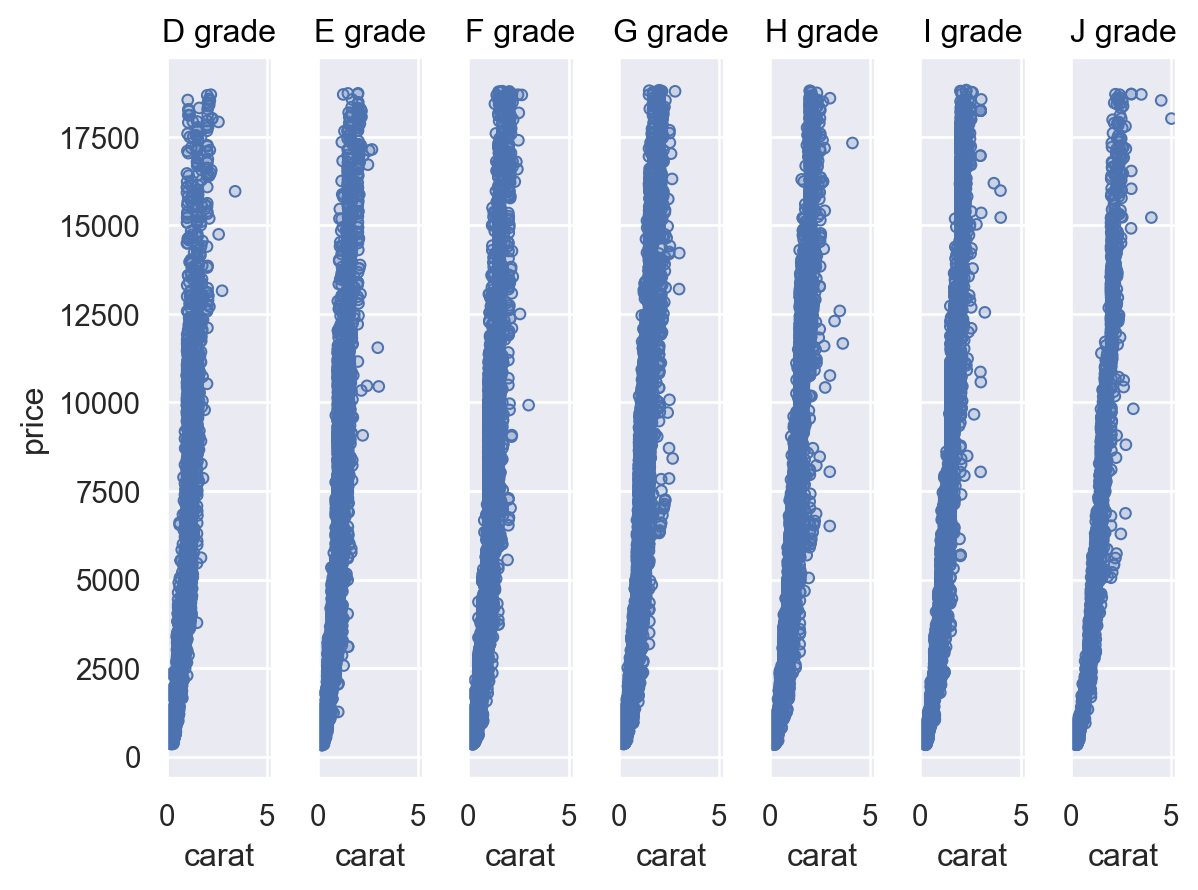# seaborn.objects.Plot.facet#

Plot.facet(col=None, row=None, order=None, wrap=None)#

Produce subplots with conditional subsets of the data.

Parameters:
col, rowdata vectors or identifiers

Variables used to define subsets along the columns and/or rows of the grid. Can be references to the global data source passed in the constructor.

orderlist of strings, or dict with dimensional keys

Define the order of the faceting variables.

wrapint

When using only `col` or `row`, wrap subplots across a two-dimensional grid with this many subplots on the faceting dimension.

Examples

Assigning a faceting variable will create multiple subplots and plot subsets of the data on each of them:

```p = so.Plot(penguins, "bill_length_mm", "bill_depth_mm").add(so.Dots())
p.facet("species")
```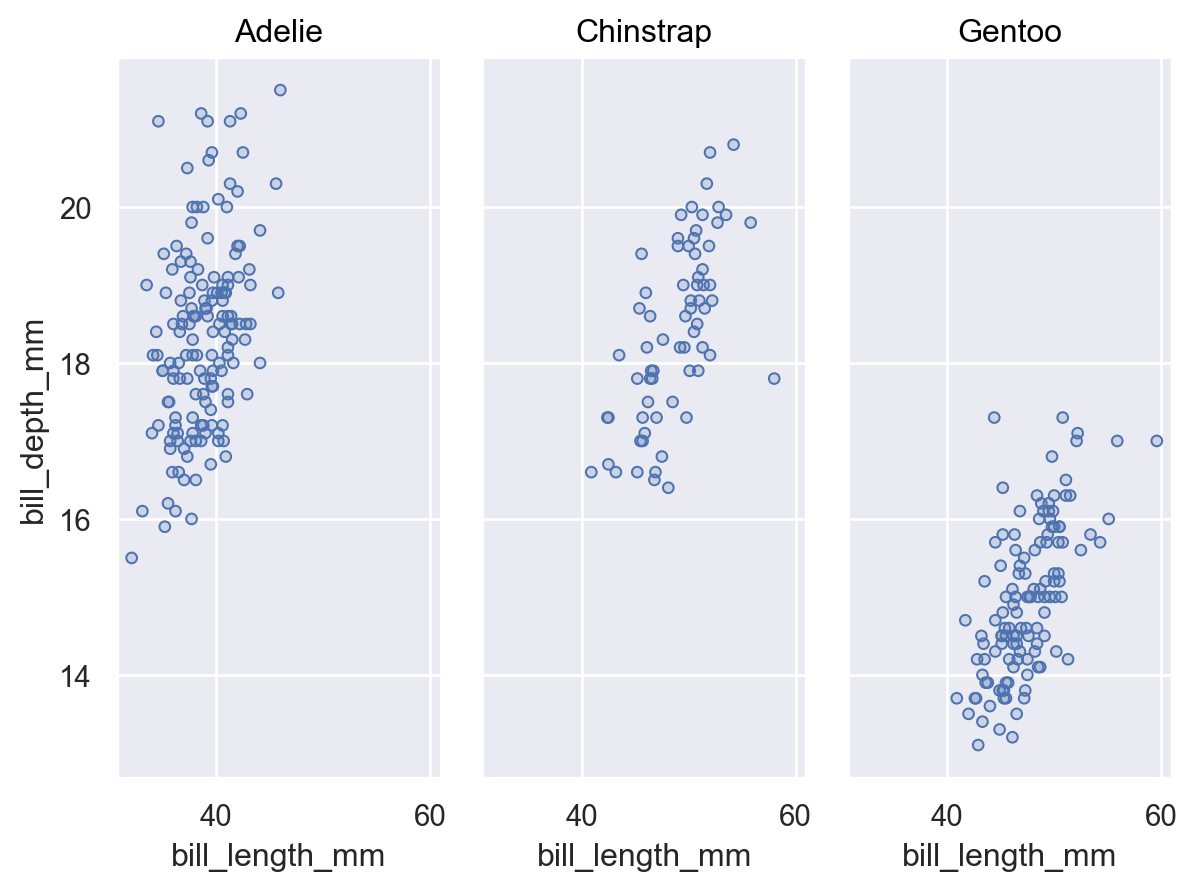Multiple faceting variables can be defined to create a two-dimensional grid:

```p.facet("species", "sex")
```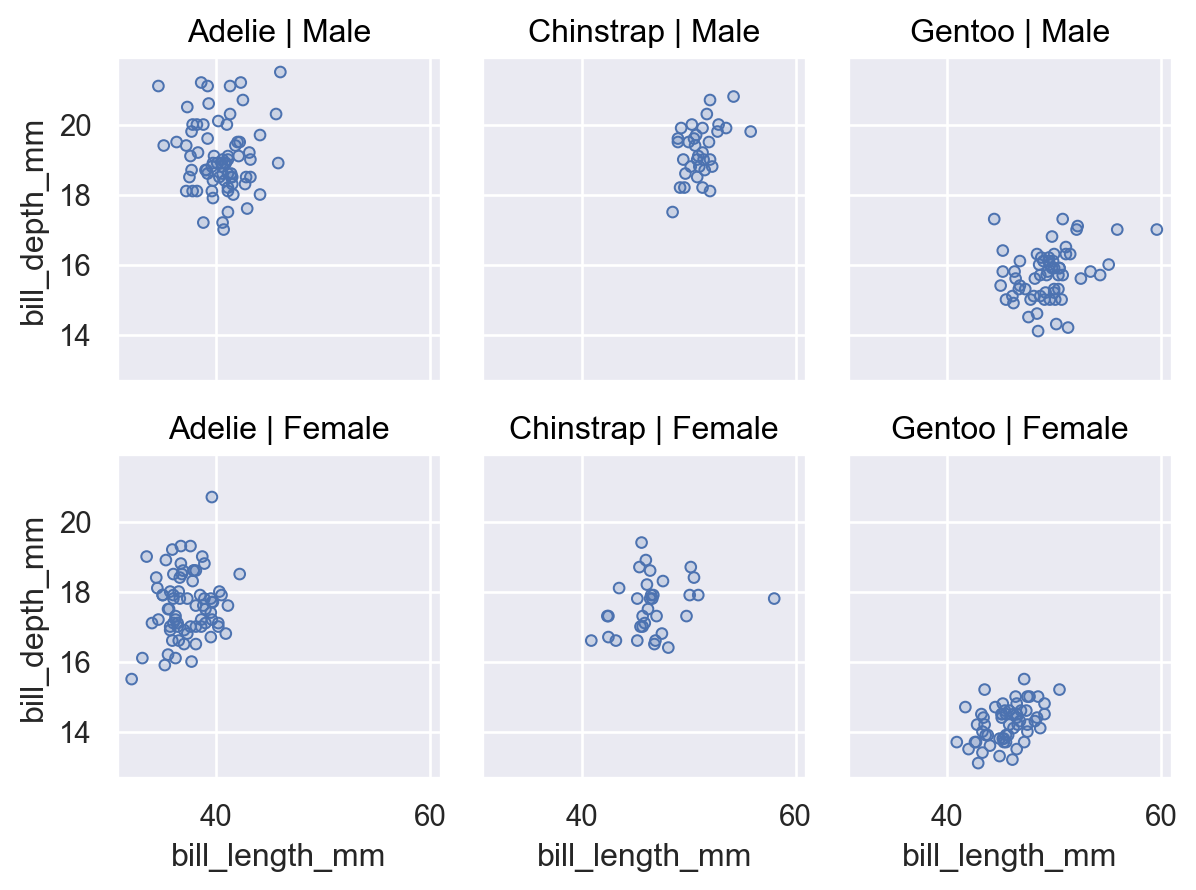Facet variables can be provided as references to the global plot data or as vectors:

```p.facet(penguins["island"])
```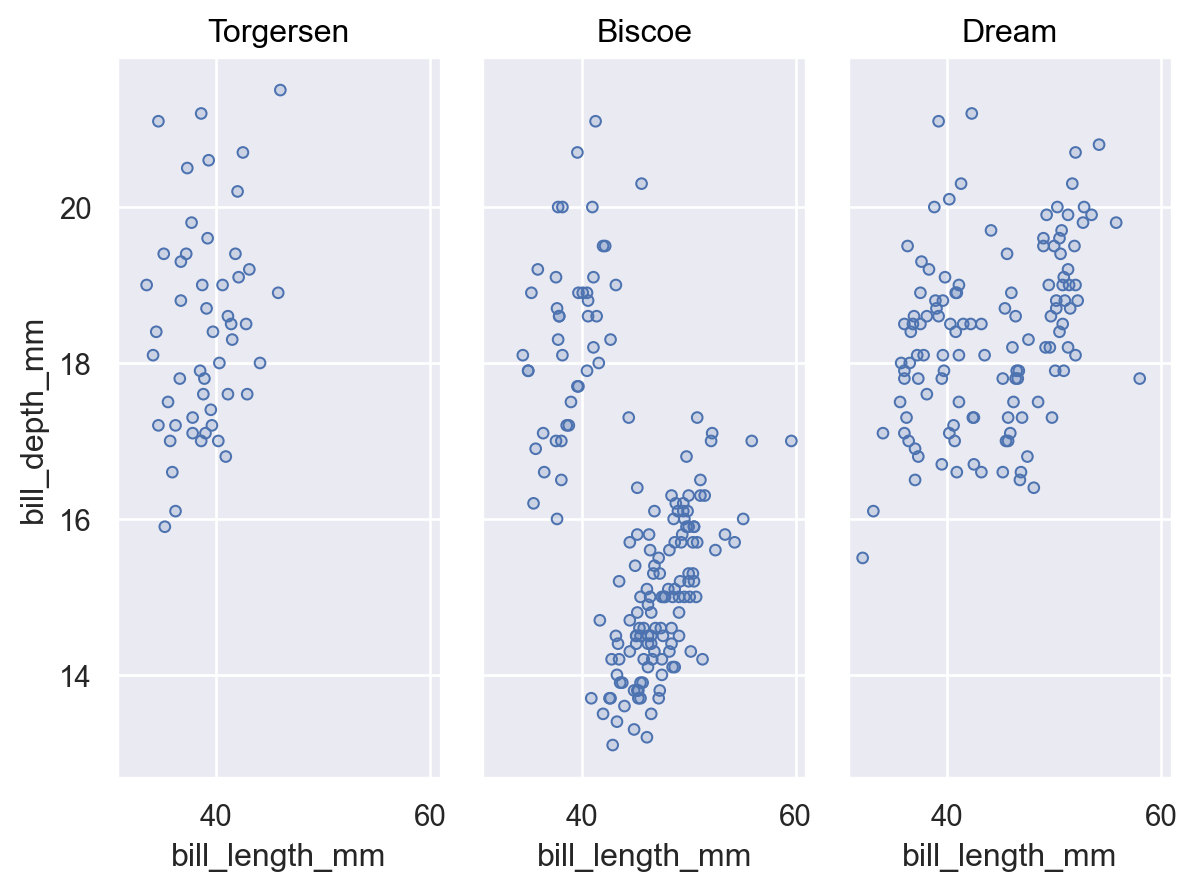With a single faceting variable, arrange the facets or limit to a subset by passing a list of levels to `order`:

```p.facet("species", order=["Gentoo", "Adelie"])
```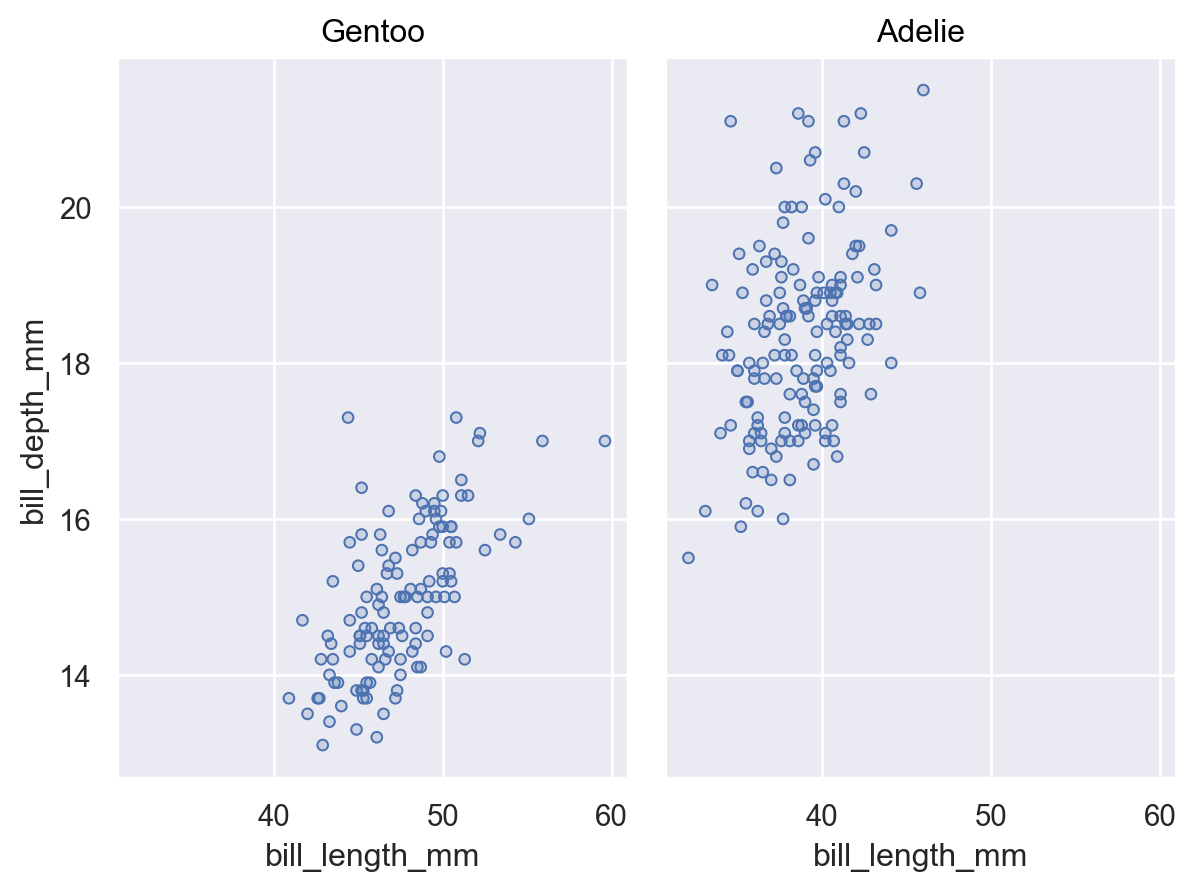With multiple variables, pass `order` as a dictionary:

```p.facet("species", "sex", order={"col": ["Gentoo", "Adelie"], "row": ["Female", "Male"]})
```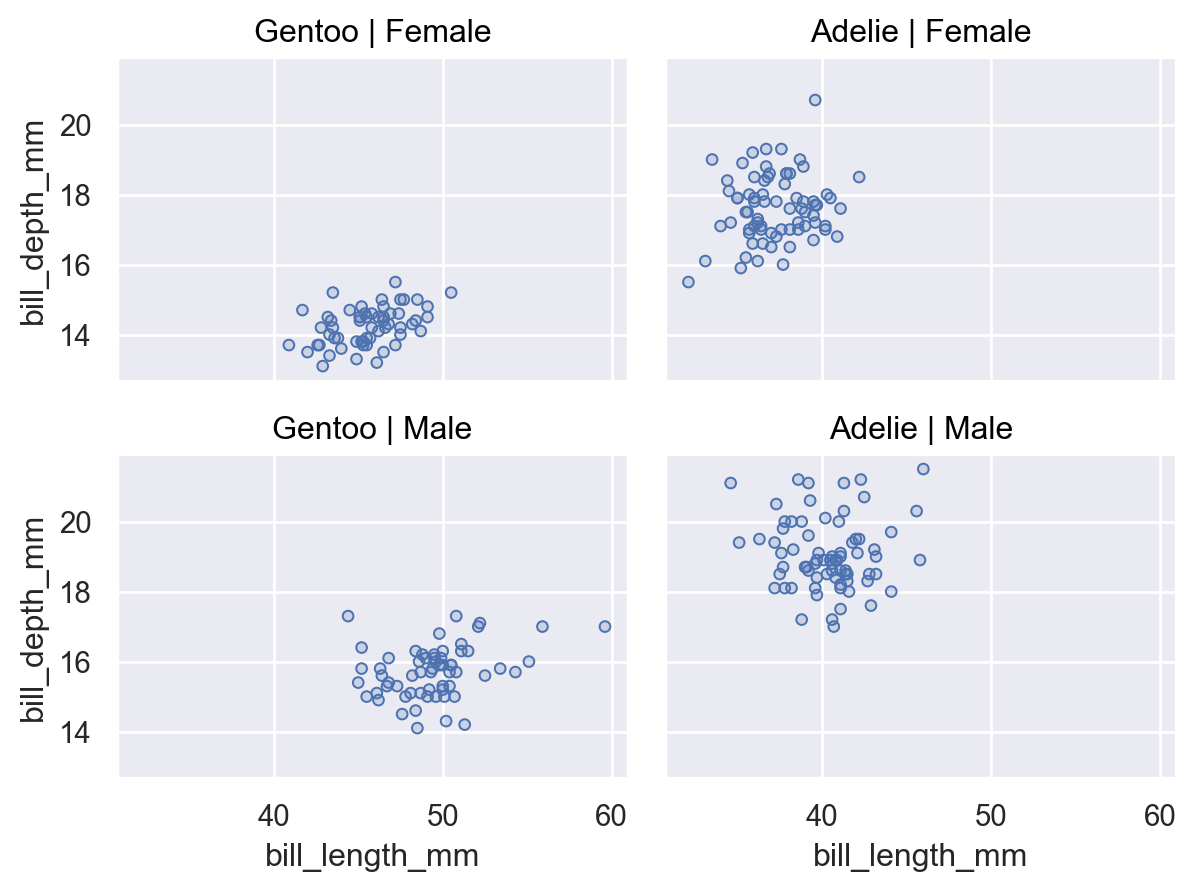When the faceting variable has multiple levels, you can `wrap` it to distribute subplots across both dimensions:

```p = so.Plot(diamonds, x="carat", y="price").add(so.Dots())
p.facet("color", wrap=4)
```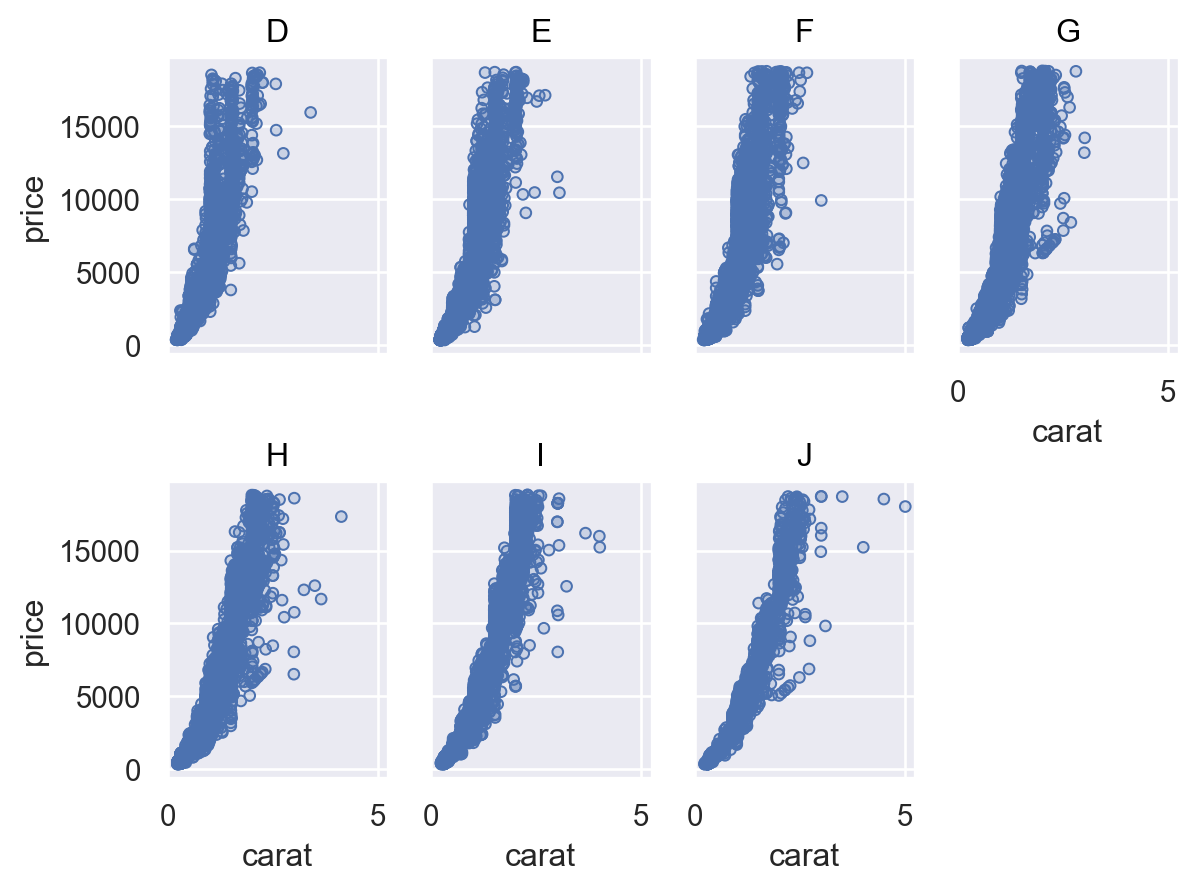Wrapping works only when there is a single variable, but you can wrap in either direction:

```p.facet(row="color", wrap=2)
```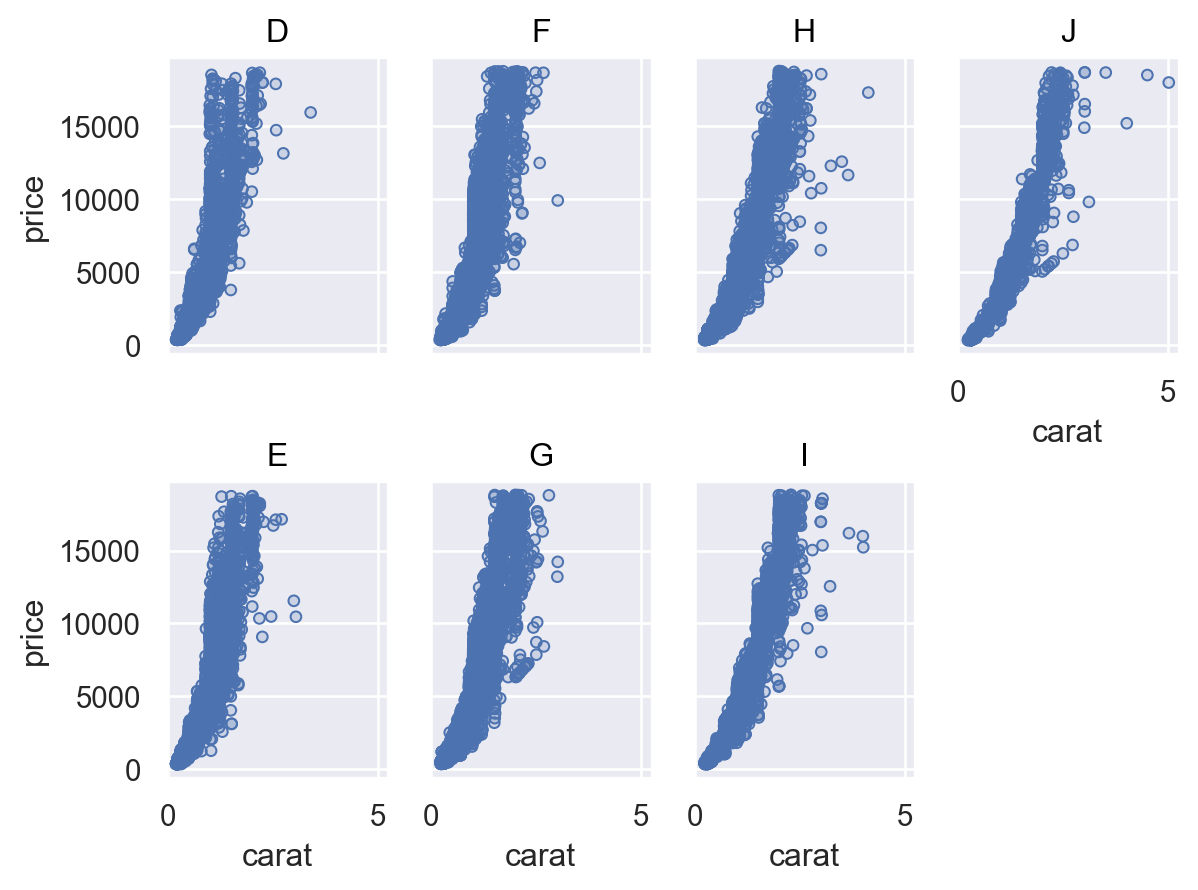Use `Plot.share()` to specify whether facets should be scaled the same way:

```p.facet("clarity", wrap=3).share(x=False)
```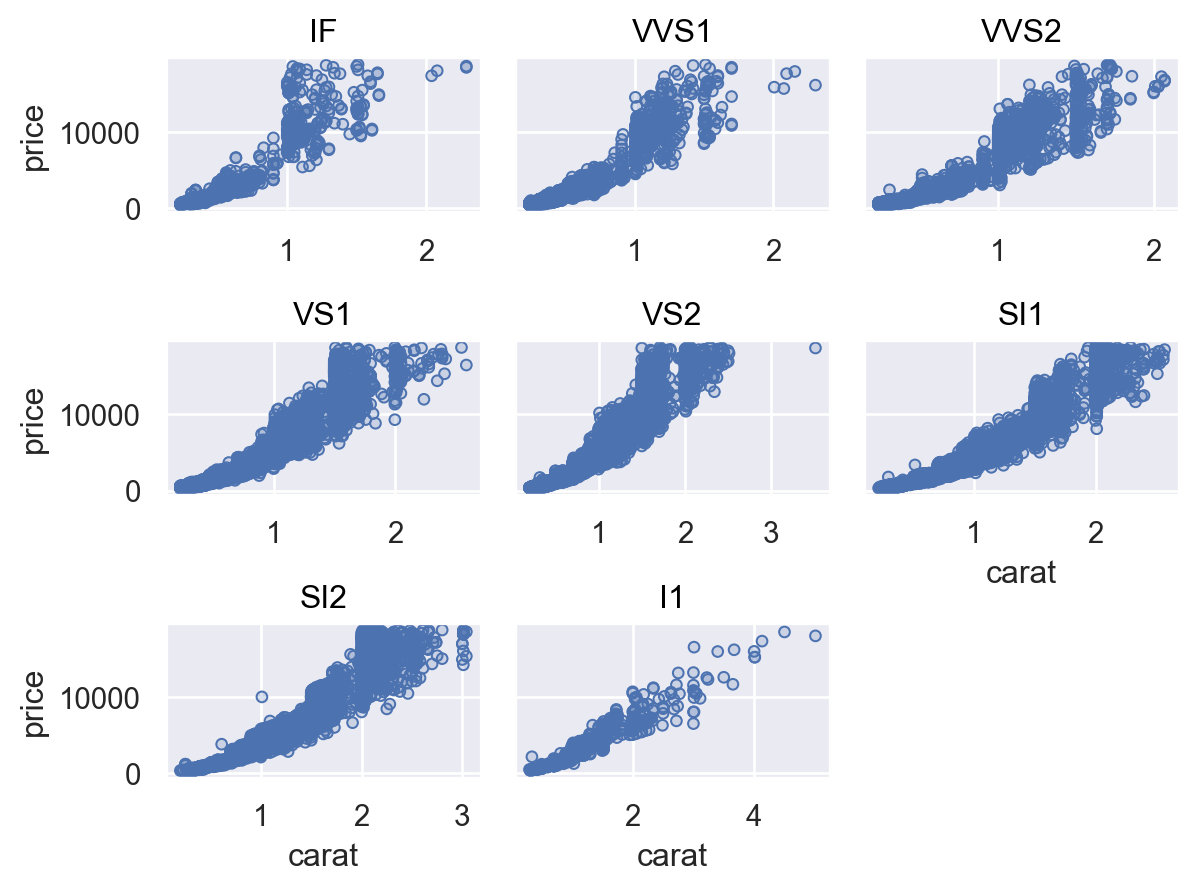Use `Plot.label()` to tweak the titles:

```p.facet("color").label(title="{} grade".format)
```